# Quantitative Aptitude Quiz For RBI Attendant 2021- 6th March

Directions (1-5): In the following questions there are two equations given. You have to solve both the equations and give answer:
(a) if x > y
(b) if x < y
(c) if x ≥ y
(d) if x ≤ y
(e) if x = y or relation between x and y cannot be established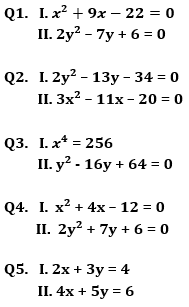Directions (6- 10): In each of these questions, two equations (I) and (II) are given. You have to solve both the equations and give answer
(a) if x>y
(b) if x≥y
(c) if x<y
(d) if x ≤y
(e) if x = y or no relation can be established between x and y.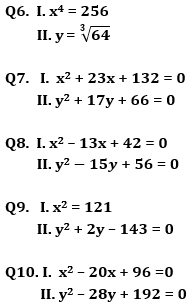Directions (11-15): In each of the following questions two equations are given. Solve these equations and give answer:
(a) if x≥y
(b) if x>y
(c) if x≤y
(d) if x<y
(e) x=y or no relation can be established between x and y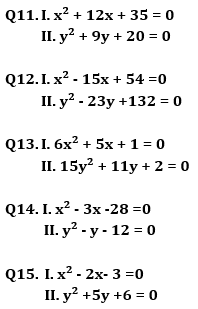Practice More Questions of Quantitative Aptitude for Competitive Exams:

###### 40 Days Study Plan for RBI Office Attendant Exam 2021

Solutions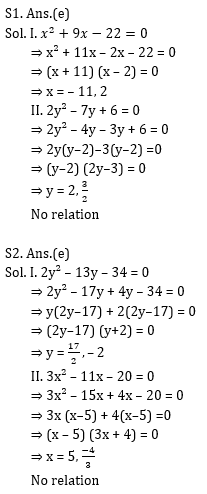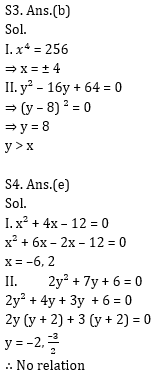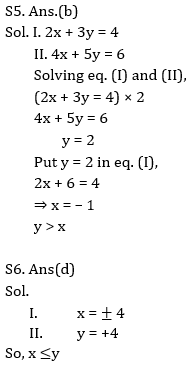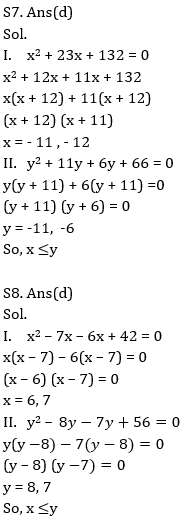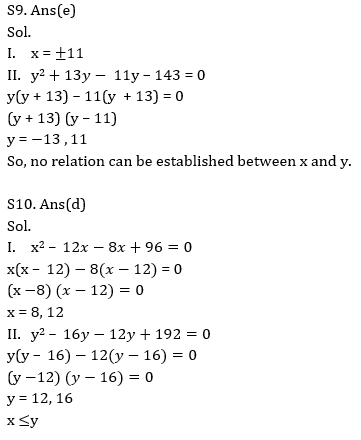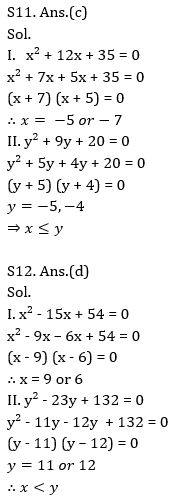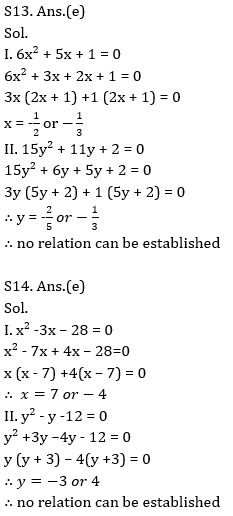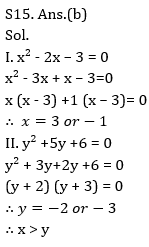Practice with Online Test Series for RBI Attendant 2021:

Click Here to Register for Bank Exams 2020 Preparation Material×

## Download success!

Thanks for downloading the guide. For similar guides, free study material, quizzes, videos and job alerts you can download the Adda247 app from play store.

Thank You, Your details have been submitted we will get back to you.
×
Login
OR

Forgot Password?

×
Sign Up
ORForgot Password
Enter the email address associated with your account, and we'll email you an OTP to verify it's you.Reset Password
Please enter the OTP sent to
/6

×
CHANGE PASSWORD